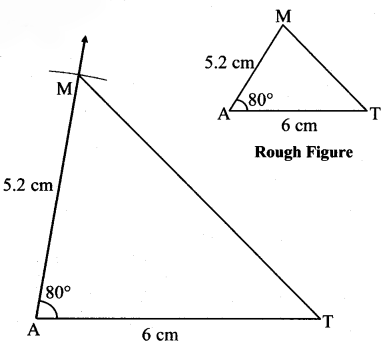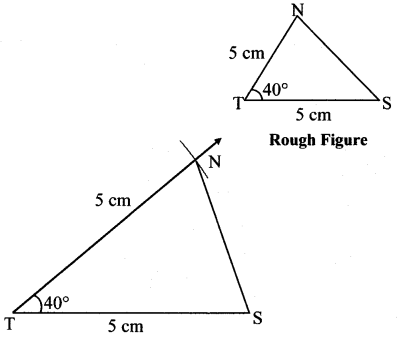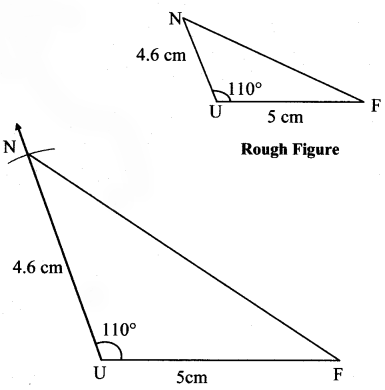# Maharashtra Board Practice Set 3 Class 7 Maths Solutions Chapter 1 Geometrical Constructions

Balbharti Maharashtra State Board Class 7 Maths Solutions covers the 7th Std Maths Practice Set 3 Answers Solutions Chapter 1 Geometrical Constructions.

## Geometrical Constructions Class 7 Practice Set 3 Answers Solutions Chapter 1

Draw triangles with the measures given below:

Question 1.
In ∆MAT, l(MA) = 5.2 cm, m∠A = 80°, l(AT) = 6 cm.
Solution:Question 2.
In ∆NTS, m∠T = 40°, l(NT) = l(TS) = 5 cm.
Solution:Question 3.
In ∆FUN, l(FU) = 5 cm, l(UN) = 4.6 cm, m∠U = 110°.
Solution:Question 4.
In ∆PRS, l(RS) = 5.5 cm, l(RP) = 4.2 cm, m∠R = 90°.
Solution: